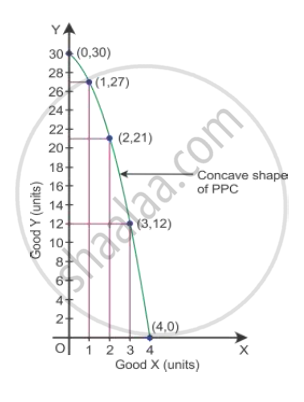# Explain the Concepts of Opportunity Cost and Marginal Rate of Transformation Using a Production Possibility Schedule Based on the Assumption that No Resource is Equally Efficient in Production of All Goods. - Economics

Explain the concepts of Opportunity Cost and Marginal Rate of Transformation using a production possibility schedule based on the assumption that no resource is equally efficient in production of all goods.

#### Solution

Marginal rate of transformation is the ratio of number of units sacrificed to gain an additional unit of another good.

MRT=(DeltaY)/(DeltaX)=

For example, assuming that resources and technology remain constant, an economy is producing Good X and Good Y. Different combinations of production of Good X and Good Y are given in the product possibility schedule:

 Production Possibilities Good X Good Y MRT=(DeltaY)/(DeltaX) I 0 30 - II 1 27 -3 III 2 21 -6 IV 3 12 -9 V 4 0 -12

In the beginning, at the production point II, where 1 unit of Good X and 27 units of Good Y are produced. To produce an additional unit of Good X, 3 units of Good Y must be sacrificed.

Here, the marginal rate of transformation (MRT) is

MRT=(DeltaY)/(DeltaX)=

Thus, MRT or the opportunity cost of getting an additional unit of Good X is 3 units of Good Y.Concept: Concept of Opportunity Cost
Is there an error in this question or solution?